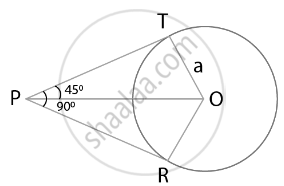# If angle between two tangents drawn from a point P to a circle of radius a and centre O is 90°, then OP = a2 - Mathematics

MCQ
True or False

If angle between two tangents drawn from a point P to a circle of radius a and centre O is 90°, then OP = asqrt(2)

• True

• False

#### Solution

This statement is True.

Explanation:

Tangent is always perpendicular to the radius at the point of contact.

Hence, ∠RPT = 90°

If 2 tangents are drawn from an external point, then they are equally inclined to the line segment joining the centre to that point.

Consider the following figure,From point P, two tangents are drawn.

It is given that, OT = a

And line OP bisects ∠RPT.

So,

∠TPO = ∠RPO = 45°

We know that, OT ⊥ PT

In right-angled triangle OTP,

Sin 45° = (OT)/(OP)

= 1/sqrt(2) = a/(OP)

Hence, OP = asqrt(2)

Concept: Concept of Circle - Centre, Radius, Diameter, Arc, Sector, Chord, Segment, Semicircle, Circumference, Interior and Exterior, Concentric Circles
Is there an error in this question or solution?

#### APPEARS IN

NCERT Mathematics Exemplar Class 10
Chapter 9 Circles
Exercise 9.2 | Q 5 | Page 105
Share